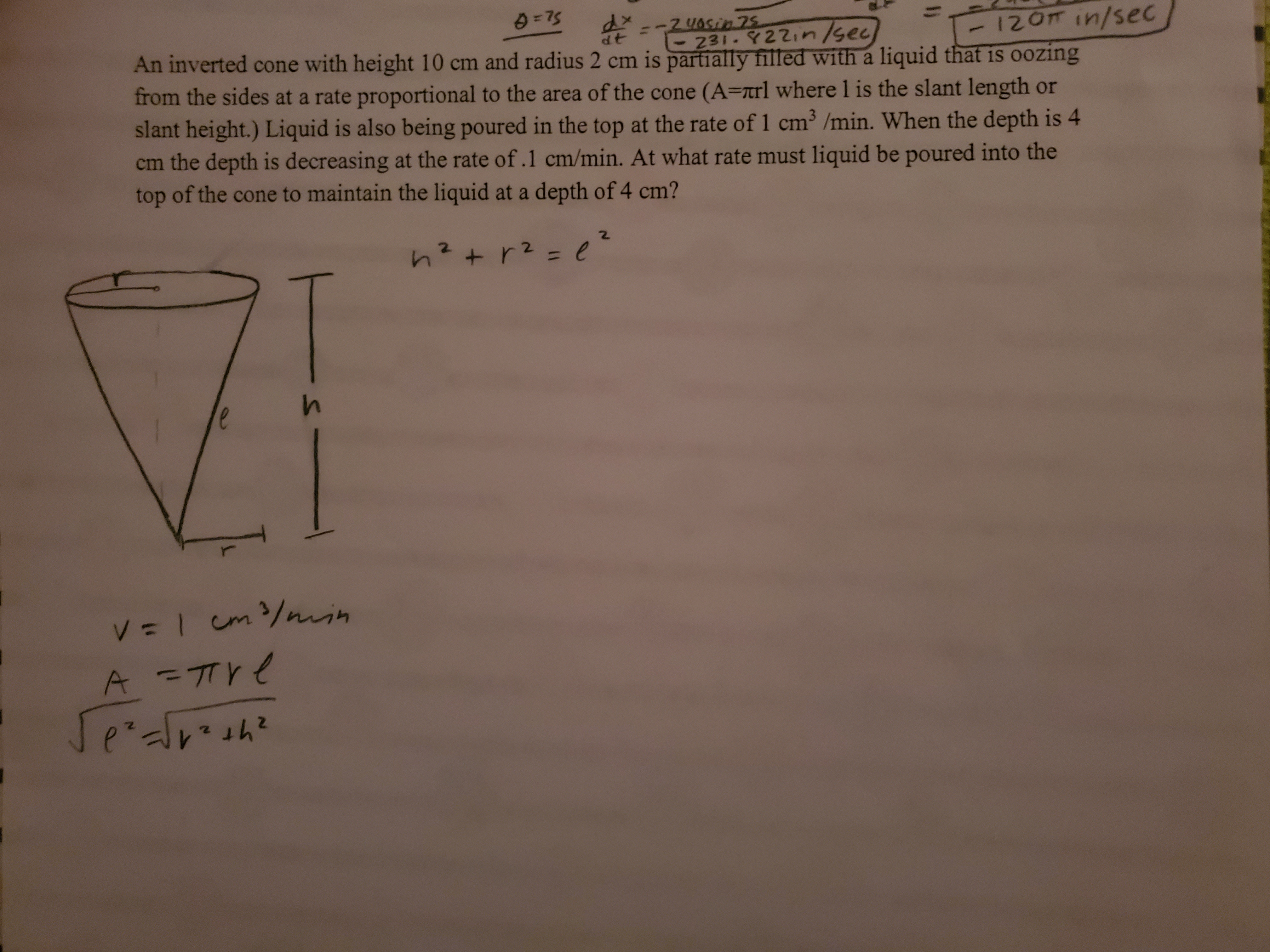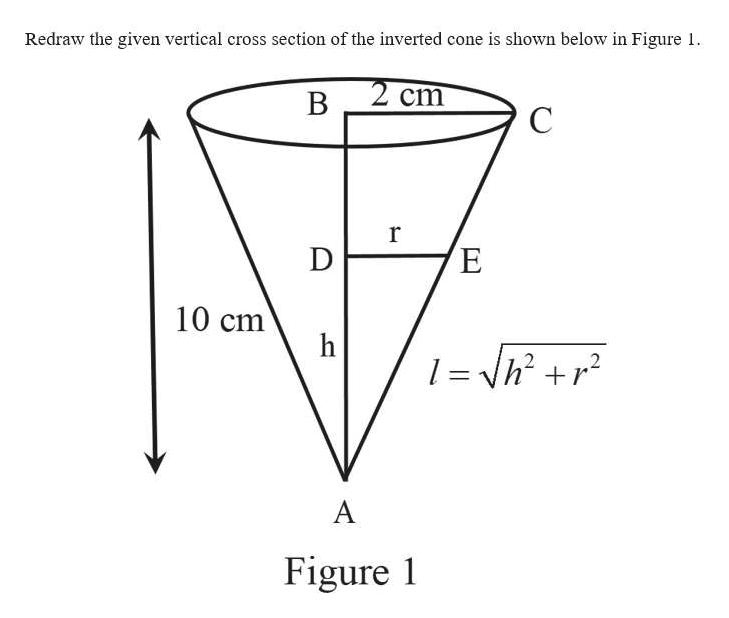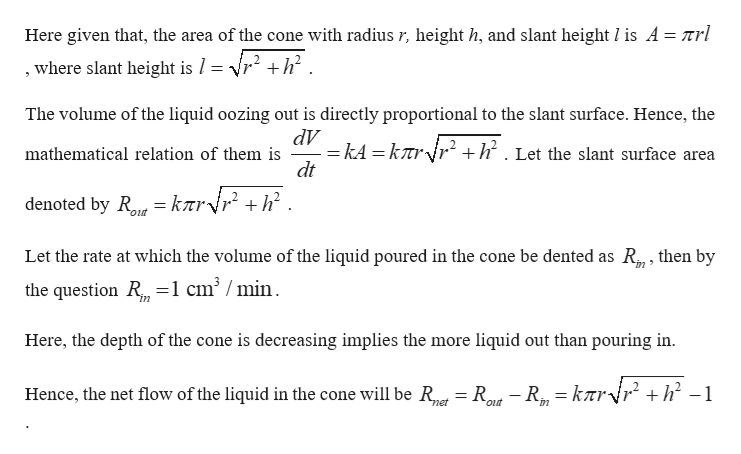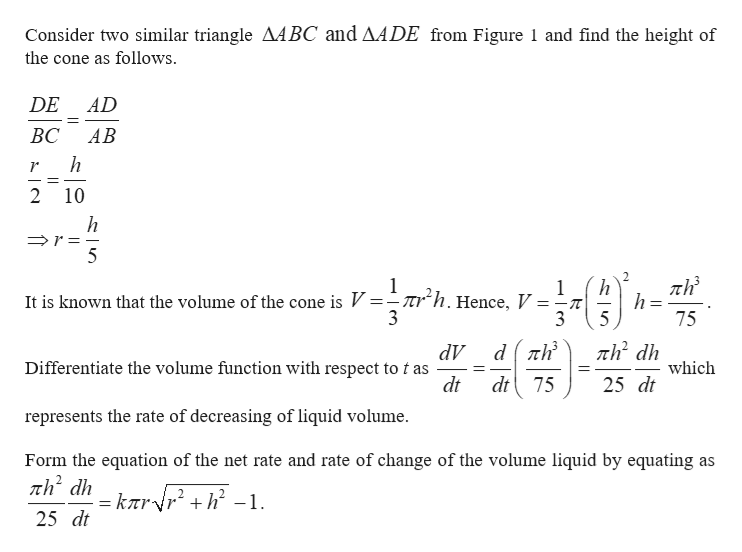# 231-22in/sec12OTIN/secAn inverted cone with height 10 cm and radius 2 cm is partially filled with a liquid that is oozingfrom the sides at a rate proportional to the area of the cone (A=7trl where l is the slant length orslant height.) Liquid is also being poured in the top at the rate of 1 cm3 /min. When the depth is 4the depth is decreasing at the rate of .1 cm/min. At what ratemust liquid be poured into thetop of the cone to maintain the liquid at a depth of 4 cm?e=1cm/nuihA -TTYZ2e2

Question
99 viewshelp_outlineImage Transcriptionclose231-22in/sec 12OTIN/sec An inverted cone with height 10 cm and radius 2 cm is partially filled with a liquid that is oozing from the sides at a rate proportional to the area of the cone (A=7trl where l is the slant length or slant height.) Liquid is also being poured in the top at the rate of 1 cm3 /min. When the depth is 4 the depth is decreasing at the rate of .1 cm/min. At what ratemust liquid be poured into the top of the cone to maintain the liquid at a depth of 4 cm? e =1cm/nuih A -TTY Z2 e 2 fullscreen
check_circle

Step 1help_outlineImage TranscriptioncloseRedraw the given vertical cross section of the inverted cone is shown below in Figure 1 2 cm В r Е D 10 cm h 2 A Figure 1 fullscreen
Step 2help_outlineImage TranscriptioncloseHere given that, the area of the cone with radius r, height h, and slant height I is A= nrl +h2 where slant height is / = ' The volume of the liquid oozing out is directly proportional to the slant surface. Hence, the dV =kA =kr{r° +h. Let the slant surface area dt mathematical relation of them is knrvr + h denoted by Rout Let the rate at which the volume of the liquid poured in the cone be dented as R, then by the question R =1 cm° /min Here, the depth of the cone is decreasing implies the more liquid out than pouring in kær fr2 +h? -1 Hence, the net flow of the liquid in the cone will be Re R-R met out fullscreen
Step 3help_outlineImage TranscriptioncloseConsider two similar triangle AABC and AADE from Figure 1 and find the height of the cone as follows DE AD ВС AB h r 2 10 h r = 5 _ 2. h h= 5 1 Ttrh.Hence, V = It is known that the volume of the cone is V: 75 th2 dh dV Differentiate the volume function with respect to t as dt which dt75 25 dt represents the rate of decreasing of liquid volume Form the equation of the net rate and rate of change of the volume liquid by equating as = kīrr + h -1 25 dt fullscreen

### Want to see the full answer?

See Solution

#### Want to see this answer and more?

Solutions are written by subject experts who are available 24/7. Questions are typically answered within 1 hour.*

See Solution
*Response times may vary by subject and question.
Tagged in

### Other